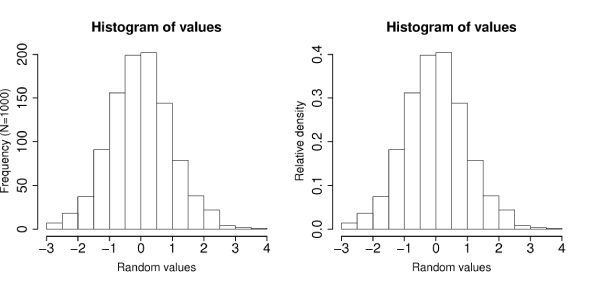# Probability Histogram Quiz: Test!

15 Questions | Total Attempts: 317SettingsDo you know what a probability histogram is? A histogram is the representation of the distribution of numerical information. To create a histogram, the first thing you must do is divide the whole range of values into a sequence of intervals and then count how many values fall into each interval. The bins must be adjacent and equal in size. Taking this quiz to test drive your knowledge of histograms would be a learning experience, good luck.

• 1.
• A.

2001-2002

• B.

2002-2003

• C.

2003-2004

• D.

2004-2005

• 2.
• A.

Bar graph

• B.

Circle graph

• C.

Histogram

• D.

Line graph

• 3.
• A.

{0, 1, 2, 4, 5, 6, 7, 7, 9, 9}

• B.

{1, 11, 23, 41, 53, 61, 71, 72, 90, 90}

• C.

{9, 9, 10, 11, 14, 16, 17, 27, 32, 35}

• D.

{27, 99, 325, 101,467}

• 4.
• A.

F

• B.

G

• C.

H

• D.

J

• 5.
• A.

The bar for 40–44 is too short and the bar for 45–49 is too tall.

• B.

The bar for 50–54 is too tall.

• C.

The bar for 50–54 is too short and the bar for 55–59 is too tall.

• D.

The bar for 55–59 is too short.

• 6.
Find the mean, median, and mode.  (Round answers to the nearest tenth.)
• A.

Mean: 54.6 Median: 55 Mode: 51

• B.

Mean: 54.6 Median: 62 Mode: 51

• C.

Mean: 55 Median: 54.6 no mode

• D.

Mean: 55 Median: 55 no mode

• 7.
• A.

The value of Q1 is incorrect

• B.

The value of the median is incorrect.

• C.

The value of Q3 is incorrect.

• D.

The boxplot is correct

• 8.
• A.

The entertainment sector is too small relative to the groceries sector

• B.

The rent sector is too large relative to the entertainment sector.

• C.

The sample size is too small.

• D.

The sectors do not add to 100%.

• 9.
What is the experimental probability that  that the spinner lands on red?
• A.

4%

• B.

20%

• C.

25%

• D.

40%

• 10.
If Angie spins the spinner 250 times,  predict the number of times it will land  on green.
• A.

10

• B.

25

• C.

50

• D.

125

• 11.
Find the theoretical probability of  randomly choosing a vowel from  the letters in EXPERIMENT
• A.
• B.
• C.
• D.
• 12.
The probability of picking a red marble from a bag is . What are the odds against picking a red marble?
• A.

2:5

• B.

5:2

• C.

5:7

• D.

7:5

• 13.
A number cube is rolled 2 times in a  row. What is the probability of rolling a  multiple of 3 both times?
• A.
• B.
• C.
• D.
• 14.
A game board has 8 cards, and 2 say  WIN. Mayela picks 2 cards without  replacing the first. What is the probability  that neither say WIN?
• A.
• B.
• C.
• D.
• 15.
From 5 players, 2 are chosen to play the  bonus round. How many different teams  of two are possible? Does the situation  involve permutations or combinations?
• A.

10; combinations

• B.

20; combinations

• C.

20; permutations

• D.

60; permutations

Related TopicsBack to top